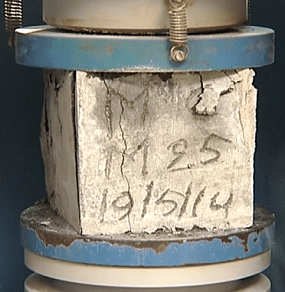# Acceptance Criteria Concrete Cube Test Results

If the cube test results show the concrete strength failure, that was an awkward moment for an Engineer. Most of the Engineers not aware of How to implement the compressive strength test of Concrete and how to evaluate it.

If we may get the failure result of concrete cube test need not worry about it! there is a way to find out does the cube was really failed or not. Sometimes we may implement the cube test wrongly or the cube was cast with more slurry it is called human error.

So now we are going to discuss what are the criteria mentioned in IS code to accept or neglect the Concrete cube test results.

Before to get into the discussion must read the Method of Concrete Test.

#### Reject the Cube Specimen when you notice the following points!.

• If it is porous or honeycomb
• Not in Even shape
• The weight of the individual cube below 7.5 Kg
• More Slurry

So avoid the cube specimen when you met the above criteria because the cube results will show failure results.

# Acceptance Criteria Concrete Cube Test Results

We well aware the Compressive strength value should be taken as the average strength of the three specimens.• The individual variation of the specimens should not be more than ± 15% of the average strength if more then the test specimens are invalid.

#### Example

For an example, If you get the three specimens Crushing Strength Value 20,25,30 respectively. Now the average compressive strength value 25 but the individual variance between the specimen is more than 15%.

Hence the Specimen sample was invalid.

The acceptance criteria have illustrated for both concrete compressive and flexural strength.

### Compressive strength

• The mean compressive strength of concrete has been considered from four consecutive test results and it should comply with the limits as shown in below table.
• The individual test result should comply with the appropriate limit as shown in below table.

### Flexural Strength

• The mean flexural strength of concrete has been considered from four consecutive test results exceeds the specified characteristic strength by at least 0.3 N/Sqmm.
• The determined flexural strength is should not be less than the compressive strength less 0.3 N/Sqmm.

We hope, you may well understand the Acceptance criteria concrete cube test results. If any doubts don’t forget to comment.

Want to know about Calculation of Standard deviation of Concrete.